# 软煤层逐级跃升水力压裂增渗技术研究Study on Increasing Permeability of Soft Coal Seams by Step-by-Step Hydraulic Fracturing Technology

• 全文下载: PDF(971KB)    PP.289-295   DOI: 10.12677/ME.2019.73040
• 下载量: 252  浏览量: 352   国家科技经费支持

Hydraulic fracturing has been widely used as a high-efficiency gas extraction and gas disaster prevention technology. The existing hydraulic fracturing technology mostly uses high-pressure pulse or low-pressure hydraulic to fracturing the coal around the borehole. This method can achieve good results in hard coal seam, but in soft coal seam, high-pressure water with large flow largely destroys the coal and rock mass around the borehole, which makes the soft coal seam destroyed or closed when the secondary cracks are not fully developed. Therefore, the application effect of hydraulic fracturing in soft coal seam is not significant, and even easy to cause the risk of coal extrusion or outburst. In the application of low-pressure hydraulic fracturing technology in soft coal seam, there are two main problems: one is that the influence scope is small, it is not easy to form differential pressure, and the fracture cannot be fully expanded; the other is that the fracturing construction time is long, the water consumption is large, and the drainage is difficult, which increases the cost and difficulty of gas control. On this issue, aimed at how to effectively extend the coal and rock mass in soft coal seam under the condition of fully developed cracks, a method of step-by-step jump fracturing hydraulic pressure and increasing coal seam permeability is put forward for the first time.

1. 引言

2. 逐级跃升水力压裂增透机理

3. 逐级跃升水力压裂裂缝扩展模型

3.1. 逐级跃升水力压裂裂缝扩展模型建立

1) 假设裂缝的横断面为长l，宽h的长矩形；俯视面为长轴l，短轴为w的长椭圆形模型(如图1所示)；水力压裂依靠高压水提供能量，对煤岩体产生拉应力破坏，当水压力大于煤岩体疲劳强度后造成煤岩体失稳破裂及其原生裂隙扩展和延伸，假设x方向为最大主应力方向，y方向为最小主应力方向，则煤岩体原始裂隙会受到高压水作用沿y方向变高，沿x方向延伸，z方向变宽。Figure 1. The schematic diagram of fracture section model

2) 煤岩体为各向均质体；压裂液为不可压缩的单相牛顿流体；压裂液在裂隙中为层流；忽略煤岩体内瓦斯气体的影响；h为裂缝高度；w为裂缝半宽度；l为压裂液扩散距离(l随时间变化而延伸l = l(t))。

3) 不考虑压裂过程中压裂液滤失量。

4) 忽略通道内的重力的影响，压裂液流动过程中受煤岩体的反向剪切力作用，摩擦阻力系数λ = 64/Re (Re为雷诺系数，取值0.6125)。

$\frac{{x}^{2}}{{l}^{2}}+\frac{{y}^{2}}{{w}^{2}}=1$ (1)

${w}_{0}=\frac{4}{E}K\sqrt{\frac{l}{\text{π}}}$ (2)

$Q=qt\approx 2h{\int }_{0}^{l}w\left(l\right)\text{d}x$ (3)

$u=\frac{\text{d}l}{\text{d}t}=\frac{qE}{3wK\sqrt{\text{π}l}}$ (4)

3.2. 逐级跃升水力压裂裂缝扩展数值模拟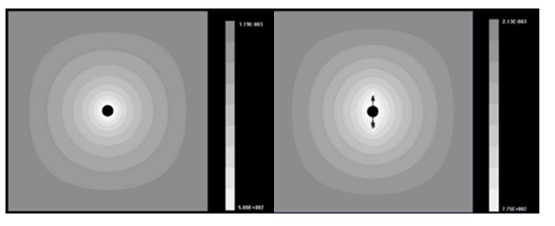Figure 2. The fracture propagation in initial hydraulic fracturing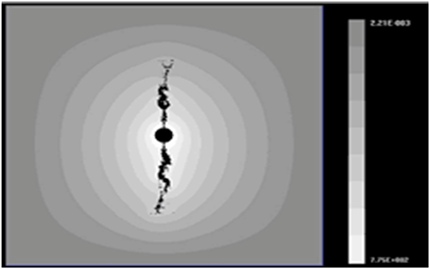Figure 3. The fracture propagation in step-by-step jump hydraulic fracturing

4. 裂缝扩展模型分析解算

$\tau =z\lambda \frac{\text{d}p}{\text{d}x}={\tau }_{s}+\mu \gamma$ (5)

$\frac{\text{d}u}{\text{d}z}=\frac{1}{\mu }\left(z\lambda \frac{\text{d}p}{\text{d}x}-{\tau }_{s}\right)$ (6)

$\begin{array}{l}{L}_{\mathrm{max}}=\left(\left(64{E}^{2}{q}^{2}{\mu }^{2}-12{\text{π}}^{\frac{1}{2}}{w}^{5}k{\lambda }^{2}\tau \left({w}^{2}-4{z}_{0}{}^{2}\right)\left({\text{π}}^{\frac{1}{2}}\Delta pk{\lambda }^{3}\left(8{z}_{0}{}^{3}-{w}^{3}\right)+8Eq\mu {l}_{0}{}^{\frac{1}{2}}\right)\\ \text{}{{-3{\text{π}}^{\frac{1}{2}}{l}_{0}{w}^{4}{\lambda }^{2}\tau \left({w}^{2}-4{z}_{0}{}^{2}\right)\right)}^{\frac{1}{2}}+8Eq\mu \right)}^{2}/\left(36\text{π}{k}^{2}{\lambda }^{4}{\tau }^{2}{\left({w}^{2}-4{z}_{0}{}^{2}\right)}^{2}\right)\end{array}$Table 1. The calculating parameter table of crack propagation model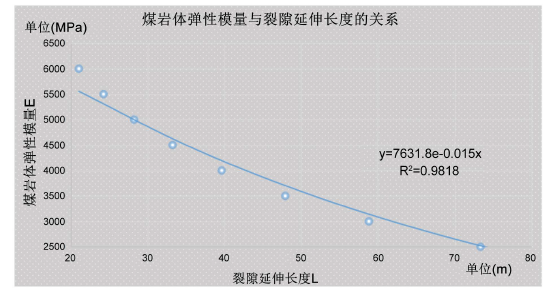Figure 4. The elastic modulus of coal and rock mass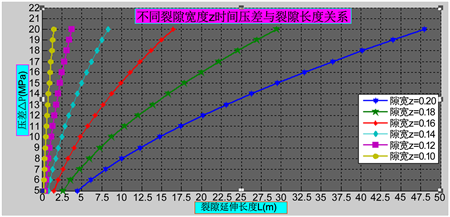Figure 5. The fracture propagation and extension relationship under different fracture width conditions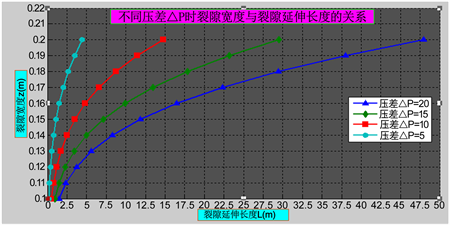Figure 6. The fracture propagation and extension relationship under different pressure difference conditions

5. 结语

1) 提出了一种逐级跃升水利压裂增渗方法，该方法既避免了高压水力直接压裂对软煤体破坏损伤，造成裂隙闭合；又避免了低压水力压裂的动力不足，压裂范围较小的缺陷，极大地提高了水力压裂增渗效果和应用范围。

2) 得出了逐级跃升水力压裂过程中每级跃升的压力与裂缝扩展长度模型。煤层具有较强的应力敏感性，在逐级跃升压裂过程中，煤层裂隙随着能量势的逐级提升而扩展，且始终沿着最大主应力方向扩展延伸，增渗效果非常明显。

3) 裂缝扩展过程中煤岩体弹性模量、逐级跃升的压差，对裂缝扩展长度影响较大，且初始裂隙宽度和裂隙高度对逐级跃升水力压裂裂隙扩展增渗效果有一定的影响。针对逐级跃升压力过程中每级压裂时间及流量控制有待下一步应用研究。

NOTES

*通讯作者。

  章敏, 王星华, 汪优, 等. Herschel-Bulkley浆液在裂隙中的扩散规律研究[J]. 岩土工程学报, 2011, 33(5): 815-820.  李宗福, 孙大发, 陈久福, 等. 水力压裂-水力割缝联合增透技术应用[J]. 煤炭科学技术, 2015, 43(10): 72-76.  卢义玉, 葛兆龙, 李晓红, 等. 脉冲射流割缝技术在石门揭煤中的应用研究[J]. 中国矿业大学学报, 2010, 39(1): 55-58+69.  孙东玲, 胡千庭, 苗法田. 煤与瓦斯突出过程中煤-瓦斯两相流的运动状态[J]. 煤炭学报, 2012, 37(3): 452-458.  Creedy, D. and Tilley, H. (2003) Coalbed Methane Extraction and Utilization. Proceedings of the Institution of Mechanical Engineers, Part A: Journal of Power and Energy, 217, 19-25. https://doi.org/10.1243/095765003321148655  Perkins, T.K. and Kern, L.R. (1961) Width of Hydraulic Fracture. Journal of Petroleum Technology, 13, 937-949. https://doi.org/10.2118/89-PA  李亭, 杨琦, 陈万钢, 等. 煤层气压裂综合滤失系数的影响因素评价[J]. 煤炭科学与技术, 2013, 41(3): 81-83.  张小东, 张鹏, 刘浩, 等. 高煤级煤储层水力压裂裂缝扩展模型研究[J]. 中国矿业大学学报, 2013, 42(4): 574-579.  王春光, 张东旭. 深部煤矿开采瓦斯综合治理技术研究[J]. 煤炭科学技术, 2013, 41(8): 11-14.  阳友奎, 肖长富. 水力压裂裂缝形态与缝内压力分布[J]. 重庆大学学报(自然科学版), 1995, 18(3): 20-26.  许露露, 崔金榜, 黄赛鹏, 等. 煤层气储层水力压裂裂缝扩展模型分析及应用[J]. 煤炭学报, 2014, 39(10): 2068-2074.  欧阳进武, 张贵金, 刘杰. 劈裂灌浆扩散机理研究[J]. 岩土工程学报, 2018, 40(7): 1328-1335.  林柏泉, 孟凡伟, 张海宾. 基于区域瓦斯治理的钻割抽一体化技术及应用[J]. 煤炭学报, 2011, 36(1): 75-79.  Osorio, J.G., Chen, H.-Y. and Teufel, L.W. (1997) Numerical Simulation of Coupled Fluid-Flow/Geomechanical Behavior of Tight Gas Reservoirs with Stress Sensitive Permeability. Latin American and Caribbean Petroleum Engineering Conference, Rio de Janeiro, Brazil, 30 August-3 September 1997, 1-15.  徐刚, 彭苏萍, 邓绪彪. 煤层气井水力压裂压力曲线分析模型及应用[J]. 中国矿业大学学报, 2011, 40(2): 174-178.  沈春明, 林柏泉, 吴海进. 高压水射流割缝及其对煤体透气性的影响[J]. 煤炭学报, 2011, 36(12): 2058-2063.